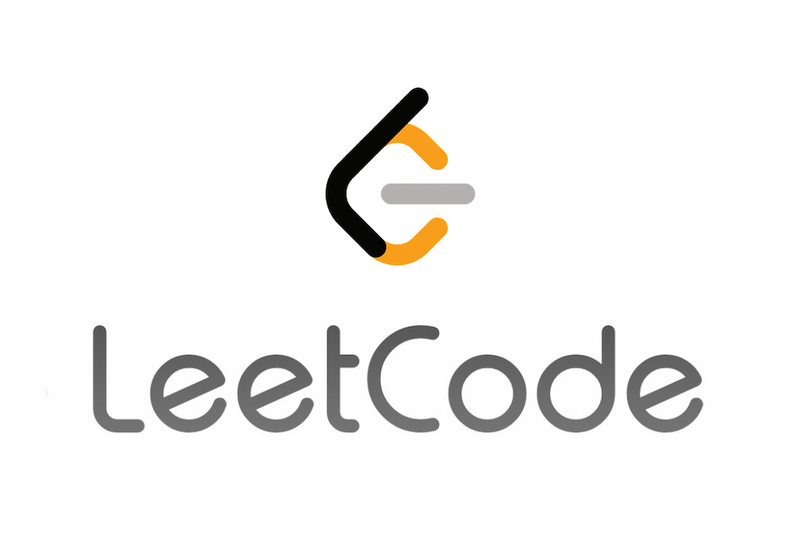# 【LeetCode】 Two Sum – 反璞歸真 – 陣列、Hash Table 雜湊表## 思路

1. 要在陣列中找到兩數之和為某數 (target)
2. 想一下小孩子會怎麼做
3. 窮舉！！！！
4. 沒錯，把每組數字都試過一遍就是了 $O(N^2)$ （解法一）
5. 我們發現不需要兩兩試過一次，a, b 握手跟 b, a 握手是一樣的，所以每一個數字只要跟後面的數字配在一起就可以，不需要回頭找配對 (解法二)
6. 上面兩個都是比較直覺的方法，解法一和解法二是從陣列裡面找答案。再想一下我們可以用 Hash Table 雜湊表來做，這樣做可以透過答案來回推
7. 以這題來說：對一個陣列裡的數字 a 而言，我們其實是在找另一個數字 target – a (解法三)

## 解法一

class Solution:
def twoSum(self, nums: List[int], target: int) -> List[int]:
for i in range(len(nums)):
for j in range(len(nums)):
if i != j:
if nums[i] + nums[j] == target:
return [i, j]

## 解法二

class Solution:
def twoSum(self, nums: List[int], target: int) -> List[int]:
for i in range(len(nums)-1):
for j in range(i+1, len(nums)):
if nums[i] + nums[j] == target:
return [i, j]

## 解法三

class Solution:
def twoSum(self, nums: List[int], target: int) -> List[int]:
ht = {}
for i in range(len(nums)):
ht[nums[i]] = i
for j in range(len(nums)):
c = target - nums[j]
if c in ht and ht[c] != j:
return [j, ht[c]]

## 筆記

• [重要！] 在 hash table (python 的 dict) 中找一個值是否有在理面的時間複雜度是 $O(1)$
• 官方解答非常值得參考，裡面也提到了更有效率的 One-pass Hash Table
https://leetcode.com/problems/two-sum/solutions/127810/two-sum/
• HackMD 筆記: https://hackmd.io/@ga642381/HJEX5yZtiGrandma